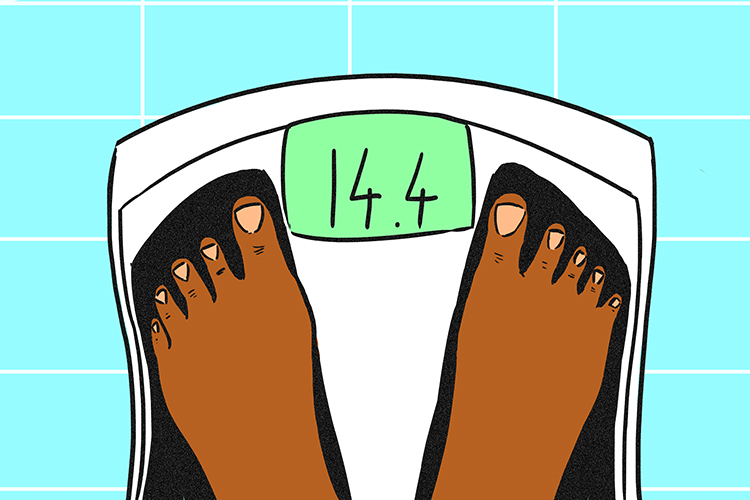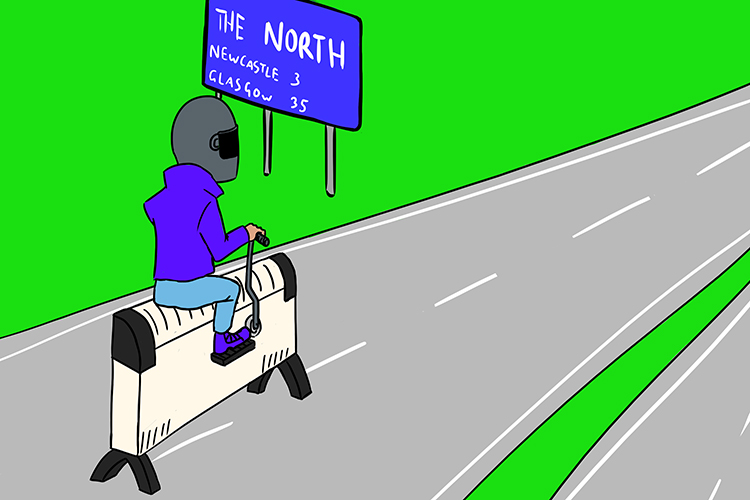# Vectors and scalars

## Scalar

Scalar – Quantity having only magnitude (not direction). It’s only a number.The scales (scalar) only give you a number.

Scalar quantities include:

• Speed
• Area
• Value
• Temperature
• Distance
• Time
• Mass

To name but a few.

All these examples only have size (magnitude). Unlike a vector, it doesn’t mention direction.

Scalars are easy to use. You just add the numbers.

Example 1

 3m2 + 4m2 = 7m2 Area + Area = Area

Example 2

A person buys a bag of sugar labelled with a mass of 500g. The mass of this bag of sugar is a scalar quantity. It only needs a number to describe it.

## Vector

Vector – Is a line which has magnitude (how long it is) and direction.They drove the convector for a long period of time (how long) northwards (and direction).

A vector is a physical quantity that has both a magnitude and a direction

Examples of vectors:

• Velocity (speed and direction)
• Displacement (distance in a given direction)
• Force (you push something with strength (magnitude) in a particular direction e.g. up a hill)
• Acceleration (is a vector quantity because it has both magnitude and direction. When an object has a positive acceleration, the acceleration occurs in the same direction as the movement of the object. When an object has a negative acceleration (it's slowing down) the acceleration occurs in the opposite direction to the movement of the object).

Example 1

A car travels at 70mph in the direction of East. We know the magnitude which is 70mph and going East is the direction. Speed (magnitude) and direction together form a vector we call velocity.

Example 2John walks north 20 metres. The direction”north” together with the distance “20 metres” is a vector called displacement.

Example 3

Vectors can be added or subtracted from each other to produce a resultant vector.If you were stood at the intersection of the x, y and z axis in the image above the person in the image is at a displacement from the tree. This displacement is equal to the tree's displacement position from you, minus the person's displacement position from you.

Example 4It does not matter which order you add vectors together. The resultant vector will be the same.

If you add vector a (blue vector) to vector b (red vector) the resultant green vector will be the same as if you added vector b (red vector) to vector a (blue vector).

Example 5An apple falls down at 10 metres per second. The direction “down” combined with the speed “10 metres per second” is a vector (this kind of vector is called velocity).